# Java Program to find quotient and remainder

In this tutorial you will learn about the Java Program to find quotient and remainder and its application with practical example.

In this tutorial, we will learn to create a Java Program to find Quotient and Remainder in Java using Java programming.

## Prerequisites

Before starting with this tutorial we assume that you are best aware of the following Java programming topics:

• Java Operators.
• Basic Input and Output function in Java.
• Class and Object in Java.
• Basic Java programming.
• If-else statements in Java.
• For loop in Java.

## Quotient and  Remainder

For find the Quotient:
we will use “/” operator.
For that we must divided by divisor  “dividend/divisor”.
Example:=> 12/4 =3 gives 3
For find the Remainder:
Same as to find the remainder we use “%”operator.
the dividend is divided by the divisor and the remainder  returned by the “%” modules operator.
Example:=> 12%4 =0 gives 0.

## Java Program to find quotient and remainder

In this program we will learn to create a Program to find Quotient and Remainder .We would first declared and initialized the required variables. Next, we would prompt user to input dividend and Divisor . Later in program we will find quotient and remainder.

## Output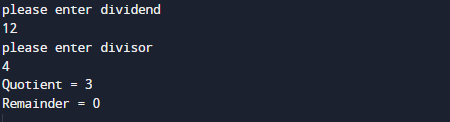In the above program, we have first declared and initialized a set variables required in the program.

• number= it will hold Dividend
• divsr= it will hold divisor.
• quotient= it will hold Quotient value.
• remainder= it will hold Remainder.

After declaring variables we going to  take value of Dividend and Divisor form user as shown in picture below.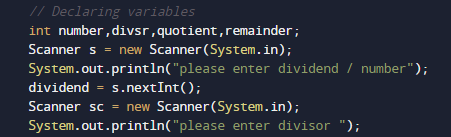After taking all the values,Now we will calculate Both Quotient and Remainder
First of all we calculate the Quotient for finding quotient we simply divide dividend by divisor using “/” operator.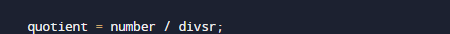then we calculate the Remainder for finding remainder we simply divide dividend by divisor using “%” operator.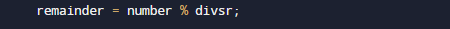At last will  print the return value by performing the operations as shown below.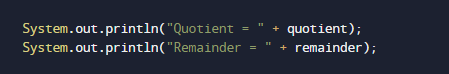In this tutorial we have learn about the Java Program to find quotient and remainder and its application with practical example. I hope you will like this tutorial.